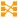# Column Mapping

 Block Group: Table Operations Icon:The Column Mapping block returns a new table that can include specified columns from the input table and can include new columns that contain calculated values.

For information on using dataflow blocks, see Dataflow.

For answers to some common questions about working with tables, see Tables.

## Input/Output Properties

The following properties of the Column Mapping block can take input and give output.

• input (table)
• retainColumns (boolean)
• name n (string)
• from n (string)

retainColumns specifies whether to include the input table's columns in the output table.

name n specifies the new column name.

from n specifies the data that will appear in the new column, using JavaScript notation. For example:

• Simple expressions: `=v1+v2` causes this column to hold the sum of the values in the v1 column and the v2 column.
• DateTime functions: `=dateFormat(v3,"y-MM-dd")` causes this column to to reformat and store the value from the v3 column.
• Number functions: `=numberFormat(v4,"0.00")` causes this column to store the value in the v4 column, formatted with the specified string.
• Conditions: `=(v5 > 1 ? true : false)` causes this column to check values in the v5 column. This example returns a TRUE boolean value if the v5 value is greater than one and a FALSE boolean value otherwise.
• Nested conditions: `=(v6 <= 1 ? false : (v6 > 2 ? false : true))` causes this column to check values in the v6 column. If the v6 value is less than or equal to one, this example returns a FALSE boolean value. Otherwise, this example returns the result of the nested condition.
• Custom math functions: `=(function(\$row) {return Math.round(Math.random() * \$row * 100);})(v7)` causes this column to perform the math function contained in this from n property and return the result. In this example, the result is the rounded product of a random number, the value in v7 for this row, and 100.
• Custom string functions: `=(function(\$row) {return \$row.length;})(v8)` causes this column to perform the string function contained in this from n property and return the result. In this example, the result is the length of the string in v8 for this row.

## Output Properties

The following properties of the Column Mapping block can give output but cannot take input.

• output (table)
• print (string)

output returns the output table with new columns.

## Storing Temporary Values

You can use `\$.<variable>` in Column Mapping and Filter to store any temporary variable between rows.

The following example returns the value of v1 from the previous row.

`=function(){var prev = \$.v1cache; \$.v1cache = v1; return prev}()`

The following example returns the difference between this row's v1 and the previous row's v1. The example converts both values to `millisecondsSinceEpoch`, then divides the difference by 60,000 to get minutes.

`=function(){var diff = (dateParse(v1) - \$.a)/1000/60; \$.a = dateParse(v1); return diff}()`

## Parsing a JSON Object

The following image shows an example. In this example, a column of the input table contains JSON. The output table contains the input JSON and also the value of the `bcd` item in the JSON for each row. See also: Scripting and Syntax.

## Examples

The following images show examples of the Column Mapping block. In the first example, the new column displays a reformatted date string. In the second example, inches are converted to centimeters.

## Use Cases

These threads in the DGLogik Community Forum show some use cases for the block: